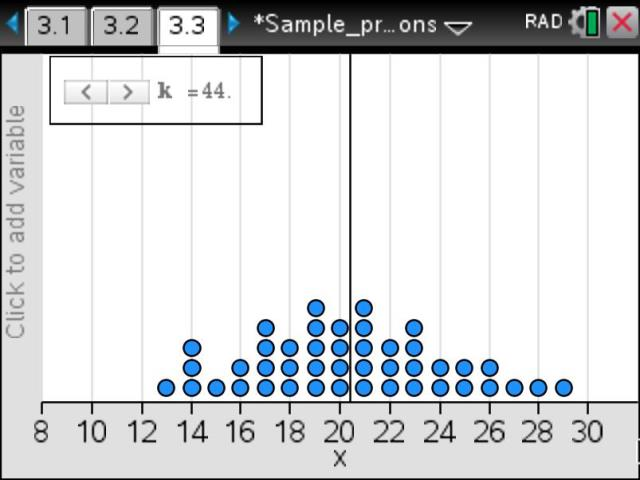# Activities

•• ##### Subject Area

• Standard: VCE: Mathematical Methods: Mathematical Methods

• ##### AuthorAust Senior

60 Minutes

• ##### Device
• TI-Nspire™
• TI-Nspire™ CAS
• TI-Nspire™ Navigator™
• TI-Nspire™ Apps for iPad®
• ##### Software

TI-Nspire™
TI-Nspire™ CAS
TI-Nspire™ CAS Navigator™ NC System
TI-Nspire™ Navigator™ NC System

4.2

• ##### Report an Issue

Introducing Sample Proportions#### Activity Overview

In this activity, simulated random sampling is used to develop the concept of the sample proportion as an estimator of the population proportion. Simulation will allow us to investigate how a sample proportion varies from sample to sample.

#### Objectives

• To appreciate variability in the results obtained from different random samples
• To investigate estimates of a population proportion from sample proportions
• To establish the idea of the sample proportion as a random variable

#### Vocabulary

population parameter, population proportion, random sample, sample statistic, sample proportion, random variable, estimation

#### About the Lesson

In this activity, simulated random sampling is used to develop the concept of the sample proportion as an estimator of the population proportion. Simulation will allow us to investigate how a sample proportion varies from sample to sample.